## A wooden block with mass 1.60 kg is placed against a compressed spring at the bottom of a slope inclined at an angle of 30.0° (point A). Whe

Question

A wooden block with mass 1.60 kg is placed against a compressed spring at the bottom of a slope inclined at an angle of 30.0° (point A). When the spring is released, it projects the block up the incline. At point B, a distance of 6.55 m up the incline from A, the block is moving up the incline at a speed of 7.50 m/s and is no longer in contact with the spring. The coefficient of kinetic friction between the block and incline is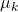= 0.50. The mass of the spring is negligible.
Calculate the amount of potential energy that was initially stored in the spring. Take free fall acceleration to be 9.80 m/s².

in progress 0
5 months 2021-08-24T22:13:03+00:00 1 Answers 33 views 0

The amount of potential energy that was initially stored in the spring is 88.8 J.

Explanation:

Given that,

Mass of block = 1.60 kg

Angle = 30.0°

Distance = 6.55 m

Speed = 7.50 m/s

Coefficient of kinetic friction = 0.50

We need to calculate the amount of potential energy

Using formula of conservation of energy between point A and B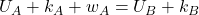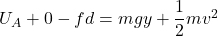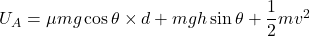Put the value into the formula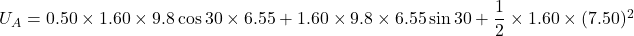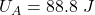Hence, The amount of potential energy that was initially stored in the spring is 88.8 J.